# Computational Models¶

The models module provides a number of published and verified computational models that can be used to predict neural responses or visual percepts resulting from electrical stimulation.

A Model object consists of:

• a SpatialModel, describing how electrical stimulation affects the neural tissue or elicited phosphene in different spatial locations of the visual field, and/or
• a TemporalModel, describing how the response of the neural tissue or elicited phosphene evolves over time.

pulse2percept provides the following computational models:

 Reference Model Type generic FadingTemporal temporal [Horsager2009] Horsager2009Model temporal [Horsager2009] Horsager2009Temporal temporal [Nanduri2012] Nanduri2012Model spatial + temporal [Nanduri2012] Nanduri2012Spatial spatial [Nanduri2012] Nanduri2012Temporal temporal [Beyeler2019] AxonMapModel spatial [Beyeler2019] ScoreboardModel spatial

Note

Spatial and temporal models can be mix-and-matched to create new models. See Creating your own model.

## Basic usage¶

All models follow the same basic work flow:

• Initialize the model with the desired model parameters.
• Build the model to perform one-time heavy computations such as building the axon map in AxonMapModel.
• Predict a percept by passing an implant that contains a stimulus. The model will return a Percept object that acts as a data container with labeled axes.

Here is how to run the ScoreboardModel:

# Initialize the model:
In : from pulse2percept.models import ScoreboardModel

In : model = ScoreboardModel(rho=200)

# Build the model:
In : model.build()
Out:
ScoreboardModel(engine='serial', grid_type='rectangular',
spatial=ScoreboardSpatial, temporal=None,
thresh_percept=0, verbose=True,
xrange=(-15, 15), xystep=0.25,
yrange=(-15, 15))

# Predict the percept resulting from stimulating Electrode
# A8 in Argus II with 30 uA:
In : from pulse2percept.implants import ArgusII

In : percept = model.predict_percept(ArgusII(stim={'A8': 30}))


To build your own model, you can mix and match spatial and temporal models at will.

For example, to create a model that combines the scoreboard model described in [Beyeler2019] with the temporal model cascade described in [Nanduri2012], use the following:

# Instantiate:
model = Model(spatial=ScoreboardSpatial(),
temporal=Nanduri2012Temporal())

# Build:
model.build()
# etc.


To create a more advanced model, you will need to subclass the appropriate base class. For example, to create a new spatial model, you will need to subclass SpatialModel and provide implementations for the following methods:

• dva2ret: a means to convert from degrees of visual angle (dva) to retinal coordinates (microns).
• ret2dva: a means to convert from retinal coordinates to dva.
• _predict_spatial: a method that accepts an ElectrodeArray as well as a Stimulus and computes the brightness at all spatial coordinates of self.grid, returned as a 2D NumPy array (space x time).

In addition, you can customize the following methods:

• __init__: the constructor can be used to define additional parameters (note that you cannot add parameters on-the-fly)
• get_default_params: all settable model parameters must be listed by this method
• _build (optional): a way to add one-time computations to the build process

A full working example:

class MySpatialModel(SpatialModel):
def __init__(self, **params):
"""Constructor"""
# Make sure to call the parent's (SpatialModel's constructor):
super(MySpatialModel, self).__init__(self, **params)
# You can set additional parameters here (e.g., stuff you will
# need later on in _build). You will not be able to add
# parameters outside the constructor or get_default_params.
self.n_fib = 100

def get_default_params(self):
"""Return a dictionary of settable model parameters"""
# Get all parameters already set by the parent (SpatialModel):
params = super(MySpatialModel, self).get_default_params()
params.update(myparam=1)
# Return the combined dictionary:
return params

def dva2ret(self, dva):
"""Convert degrees of visual angle (dva) into retinal coords (um)"""
return 280.0 * dva

def ret2dva(self, ret):
"""Convert retinal corods (um) to degrees of visual angle (dva)"""
return ret / 280.0

def _build(self):
"""Perform heavy computations during the build process"""
# Perform some expensive computation using parameters you
# initialized in the constructor:
self.heavy = some_heavy_comp(self.n_fib)

def _predict_spatial(self, earray, stim):
"""Calculate the spatial response at different time points"""
resp = np.zeros(self.grid.size, stim.time.size)
for idx_t, t in enumerate(stim.time):
for idx_xy, (x, y) in enumerate(self.grid):
# Response at (x,y,t) is the sum of x,y coordinates and
# all the stimuli at time t (an arbitrary, silly choice):
resp[idx_xy, idx_t] = x + y + np.sum(stim[:, t])
return resp


Similarly, a new temporal model needs to subclass from TemporalModel and provide a _predict_temporal method:

class MyTemporalModel(TemporalModel):
def _predict_temporal(self, stim, t_percept):
"""Calculates the temporal response at different time points"""
# Response at (x,y,t) is the stimulus at (x,y,t). Use stim's smart
# indexing to do automatic interpolation:
return stim[:, t_percept]


## Stand-alone models vs. spatial/temporal model components¶

In general, you will want to work with Model objects, which provide all the necessary glue between a spatial and/or a temporal model component. Objects are named accordingly:

However, nobody stops you from instantiating a spatial or temporal model directly:

# Option 1 (preferred): Work with Model objects:
from pulse2percept.models import Model, Nanduri2012Temporal
model = Model(temporal=Nanduri2012Temporal())
model.build()
model.predict_percept(implant)

# Option 2: Work directly with a temporal model:
model = Nanduri2012Temporal()
model.build()
model.predict_percept(implant.stim)


The differences between the two are subtle:

## Getting and setting parameters¶

A Model will hide the complexity that some parameters exist only in the spatial or temporal model component.

Consider the following model:

In : from pulse2percept.models import (Model, ScoreboardSpatial,
...:                                   Nanduri2012Temporal)
...:

In : model = Model(spatial=ScoreboardSpatial(),
...:               temporal=Nanduri2012Temporal())
...:

# Set rho param of the scoreboard model (works even though it's really
# model.spatial.rho):
In : model.rho = 123

# Print the simulation time step of the Nanduri model (works even though
# it's really model.temporal.dt):
In : print(model.dt)
0.005


Although rho exists only in the scoreboard model, and dt exists only in the temporal model, you can get and set them as if they were part of the main model.

Warning

If a parameter exists in both spatial and temporal models (e.g., thresh_percept), then calling model.thresh_percept = 0 will update both the spatial and temporal model.

Alternatively, use model.spatial.thresh_percept = 0 or model.temporal.thresh_percept = 0.

## Examples using Model¶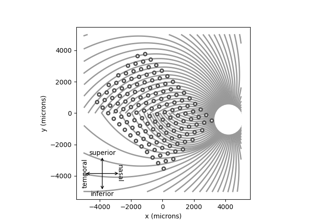Creating a grid of electrodes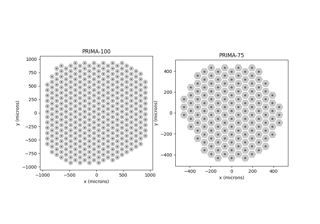Retinal implant gallery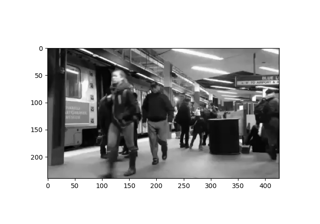Generating a stimulus from a video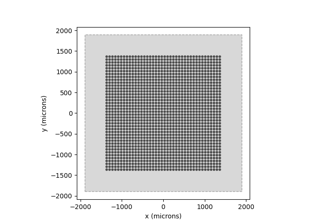Generating a stimulus from an image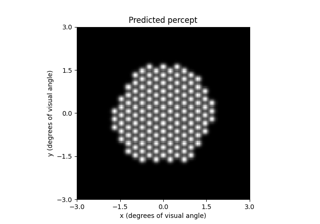Beyeler et al. (2019): Focal percepts with the scoreboard model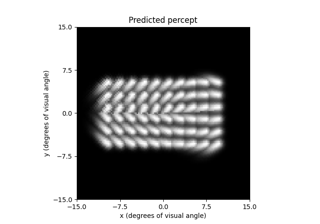Beyeler et al. (2019): Axonal streaks with the axon map modelNanduri et al. (2012): Frequency vs. amplitude modulation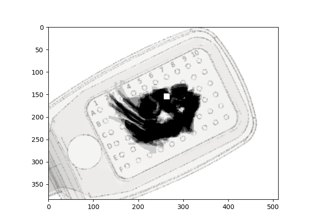Phosphene drawings from Beyeler et al. (2019)